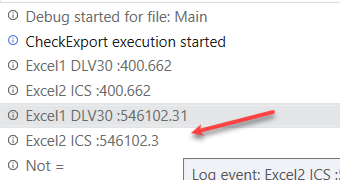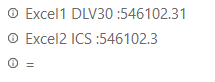# Compare the approximate value 0.05

Hello all,

I have to compare 2 cell approximate value 0.05.

in_DataTableDLV030.Rows(0)(7).ToString= in_DtPLKH.Rows(1)(11).ToString AND in_DataTableDLV030.Rows(0)(8).ToString= in_DtPLKH.Rows(2)(11).ToStringExample 546102.31 & 546102.3 not equal. How can I do compare approximate value 0.05
→ 546102.31 = 546102.3

Thank all

HI,

How about using Math.Round as the following?

``````Math.Round(546102.31,1,MidpointRounding.AwayFromZero) =Math.Round(546102.3,1,MidpointRounding.AwayFromZero)
``````

Regards,

1 Like

How I use variable for 2cell of 2 file excel:

Math.Round(in_DataTableDLV030.Rows(0)(7).ToString,1,MidpointRounding.AwayFromZero) =Math.Round(in_DtPLKH.Rows(1)(11).ToString,1,MidpointRounding.AwayFromZero) And Math.Round(in_DataTableDLV030.Rows(0)(8).ToString,1,MidpointRounding.AwayFromZero) =Math.Round(in_DtPLKH.Rows(2)(11).ToString,1,MidpointRounding.AwayFromZero)

Hi,

Can you try to use Double.Parse ( or CDbl) to convert string to double, as the following?

``````Math.Round(Double.Parse(in_DataTableDLV030.Rows(0)(7).ToString),1,MidpointRounding.AwayFromZero) =Math.Round(Double.Parse(in_DtPLKH.Rows(1)(11).ToString),1,MidpointRounding.AwayFromZero) AndAlso Math.Round(Double.Parse(in_DataTableDLV030.Rows(0)(8).ToString),1,MidpointRounding.AwayFromZero) =Math.Round(Double.Parse(in_DtPLKH.Rows(2)(11).ToString),1,MidpointRounding.AwayFromZero)
``````

Regards,

1 Like

Thank you, it run okithis is approximate value 0.05 ? if i want change 0.09 ?

HI,

The above expression is rounded to one decimal place.
If your requirement is that difference b/w 2 values is less than 0.05 (or 0.09), the following will work.

``````Math.Abs(Double.Parse(in_DataTableDLV030.Rows(0)(7).ToString) -Double.Parse(in_DtPLKH.Rows(1)(11).ToString))<0.05
``````

Regards,

1 Like

This topic was automatically closed 3 days after the last reply. New replies are no longer allowed.